Next: Experimental results Up: Extensions to the SVM Previous: Multiclass SVMs   Contents   Index

## Nonlinear SVMs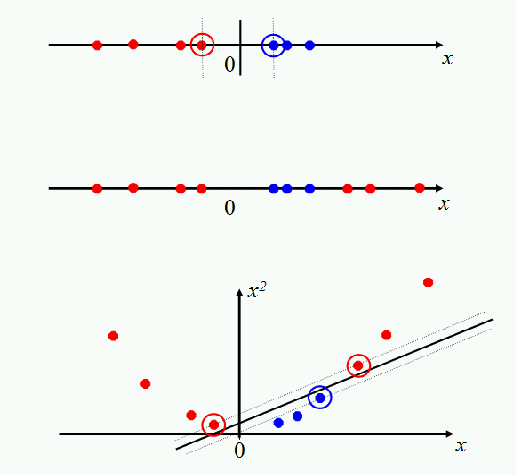With what we have presented so far, data sets that are linearly separable (perhaps with a few exceptions or some noise) are well-handled. But what are we going to do if the data set just doesn't allow classification by a linear classifier? Let us look at a one-dimensional case. The top data set in Figure 15.6 is straightforwardly classified by a linear classifier but the middle data set is not. We instead need to be able to pick out an interval. One way to solve this problem is to map the data on to a higher dimensional space and then to use a linear classifier in the higher dimensional space. For example, the bottom part of the figure shows that a linear separator can easily classify the data if we use a quadratic function to map the data into two dimensions (a polar coordinates projection would be another possibility). The general idea is to map the original feature space to some higher-dimensional feature space where the training set is separable. Of course, we would want to do so in ways that preserve relevant dimensions of relatedness between data points, so that the resultant classifier should still generalize well.

SVMs, and also a number of other linear classifiers, provide an easy and efficient way of doing this mapping to a higher dimensional space, which is referred to as the kernel trick ''. It's not really a trick: it just exploits the math that we have seen. The SVM linear classifier relies on a dot product between data point vectors. Let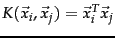. Then the classifier we have seen so far is: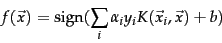(172)

Now suppose we decide to map every data point into a higher dimensional space via some transformation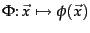. Then the dot product becomes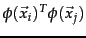. If it turned out that this dot product (which is just a real number) could be computed simply and efficiently in terms of the original data points, then we wouldn't have to actually map from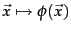. Rather, we could simply compute the quantity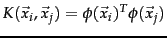, and then use the function's value in Equation 172. A kernel function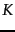is such a function that corresponds to a dot product in some expanded feature space.

Worked example. The quadratic kernel in two dimensions.quad-kernel For 2-dimensional vectors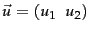,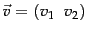, consider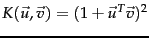. We wish to show that this is a kernel, i.e., that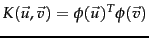for some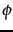. Consider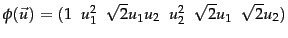. Then: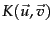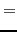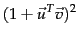(173)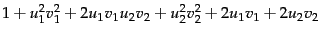(174)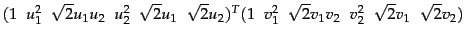(175)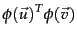(176)

End worked example.

In the language of functional analysis, what kinds of functions are valid kernel functions ? Kernel functions are sometimes more precisely referred to as Mercer kernels , because they must satisfy Mercer's condition: for any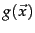such that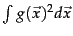is finite, we must have that: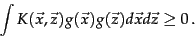(177)

A kernel functionmust be continuous, symmetric, and have a positive definite gram matrix. Such ameans that there exists a mapping to a reproducing kernel Hilbert space (a Hilbert space is a vector space closed under dot products) such that the dot product there gives the same value as the function. If a kernel does not satisfy Mercer's condition, then the corresponding QP may have no solution. If you would like to better understand these issues, you should consult the books on SVMs mentioned in Section 15.5 . Otherwise, you can content yourself with knowing that 90% of work with kernels uses one of two straightforward families of functions of two vectors, which we define below, and which define valid kernels.

The two commonly used families of kernels are polynomial kernels and radial basis functions. Polynomial kernels are of the form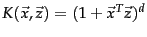. The case of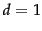is a linear kernel, which is what we had before the start of this section (the constant 1 just changing the threshold). The case of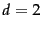gives a quadratic kernel, and is very commonly used. We illustrated the quadratic kernel in quad-kernel.

The most common form of radial basis function is a Gaussian distribution, calculated as: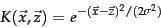(178)

A radial basis function (rbf) is equivalent to mapping the data into an infinite dimensional Hilbert space, and so we cannot illustrate the radial basis function concretely, as we did a quadratic kernel. Beyond these two families, there has been interesting work developing other kernels, some of which is promising for text applications. In particular, there has been investigation of string kernels (see Section 15.5 ).

The world of SVMs comes with its own language, which is rather different from the language otherwise used in machine learning. The terminology does have deep roots in mathematics, but it's important not to be too awed by that terminology. Really, we are talking about some quite simple things. A polynomial kernel allows us to model feature conjunctions (up to the order of the polynomial). That is, if we want to be able to model occurrences of pairs of words, which give distinctive information about topic classification, not given by the individual words alone, like perhaps operating and system or ethnic and cleansing, then we need to use a quadratic kernel. If occurrences of triples of words give distinctive information, then we need to use a cubic kernel. Simultaneously you also get the powers of the basic features - for most text applications, that probably isn't useful, but just comes along with the math and hopefully doesn't do harm. A radial basis function allows you to have features that pick out circles (hyperspheres) - although the decision boundaries become much more complex as multiple such features interact. A string kernel lets you have features that are character subsequences of terms. All of these are straightforward notions which have also been used in many other places under different names.Next: Experimental results Up: Extensions to the SVM Previous: Multiclass SVMs   Contents   Index